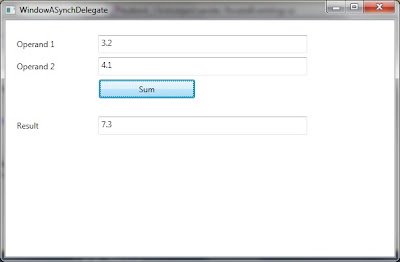## Saturday, December 11, 2010

### WPF - ASynchronous Delegate Exception Model

In this post, we are going to discuss exception model of Asynchronous delegates. For those who have started development just starting with .net 4.0, they are old school for Task. Basically thread (including ThreadPool.QueueUserWorkItem based threads) are for providing "shoot and run" kind of flows. They don't us allow to return anything from them. If we needed to return a value, the only option we have is ASynchronous Delegate. It must be remembered that Asynchronous delegate would still be using ThreadPool thread so it will have all the benefits of using a ThreadPool thread.

Like BackgroundWorker, ASynchDelegate also has a special mechanism for dealing with exceptions raised during its operation. It is necessary to be aware of it. We will be discussing the use of ASynchDelegate in a sample WPF application. In this application, we will be taking input of two operands. The application would perform some complex calculation on these operands and display the result on the form. In order to keep the example simple, we would just sum these operands. We will not be verifying the inputs just for the same reason.The XAML for the above display is as follows:
`<Window x:Class="WpfApplication_ASynchDelegate.MainWindow"        xmlns="http://schemas.microsoft.com/winfx/2006/xaml/presentation"        xmlns:x="http://schemas.microsoft.com/winfx/2006/xaml"        Title="WindowASynchDelegate" Height="389" Width="595">    <Grid>        <TextBox Height="27" HorizontalAlignment="Left" Margin="138,22,0,0"                 Name="textBoxOperand1" VerticalAlignment="Top" Width="311" />        <TextBox Height="27" HorizontalAlignment="Left" Margin="138,55,0,0"                 Name="textBoxOperand2" VerticalAlignment="Top" Width="311" />        <TextBox Height="27" HorizontalAlignment="Left" Margin="138,143,0,0"                 Name="textBoxResult" VerticalAlignment="Top" Width="311" />        <Button Content="Sum" Height="28" HorizontalAlignment="Left" Margin="139,88,0,0"                Name="button1" VerticalAlignment="Top" Width="143" Click="button1_Click" />        <Label Content="Operand 1" Height="27" HorizontalAlignment="Left" Margin="12,22,0,0"               Name="label1" VerticalAlignment="Top" Width="120" />        <Label Content="Operand 2" Height="27" HorizontalAlignment="Left" Margin="12,55,0,0"               Name="label2" VerticalAlignment="Top" Width="120" />        <Label Content="Result" Height="27" HorizontalAlignment="Left" Margin="12,143,0,0"               Name="label3" VerticalAlignment="Top" Width="120" />    </Grid></Window>`

The code behind of the above window is as follows:
`public partial class MainWindow : Window{    public MainWindow()    {        InitializeComponent();    }    private decimal getSum(decimal operand1, decimal operand2)    {        decimal sum;        sum = operand1 + operand2;        return sum;    }    private void button1_Click(object sender, RoutedEventArgs e)    {        decimal operand1 = Decimal.Parse(this.textBoxOperand1.Text);        decimal operand2 = Decimal.Parse(this.textBoxOperand2.Text);                Func<decimal, decimal, decimal> getSumAsynchDelegate = getSum;                getSumAsynchDelegate.BeginInvoke(operand1, operand2,            (resultASynch) =>            {                var methodDelegate = (Func<decimal, decimal, decimal>)resultASynch.AsyncState;                this.Dispatcher.BeginInvoke(                    new Action(() =>                               this.textBoxResult.Text =                               methodDelegate.EndInvoke(resultASynch).ToString()));            },            getSumAsynchDelegate);    }}`

In the Click event handler for the button, we have assigned the text entered in two input text boxes texBoxOperand1 and textBoxOperand2 to local variables operand1 and operand2. We have introduced an asynchronous delegate. This is expected to receive two decimal arguments and returns a decimal value. In the Asynchronous delegate declaration, the last value specified is the return type.
`Func<decimal, decimal, decimal> getSumAsynchDelegate = getSum;`

Although Asynchronous delegate seems to be similar to the Asynchronous methods (Asynchronous Programming Model - APM) because It has BeginInvoke and EndInvoke mechanism as in ASynchronous method and BeginInvoke returns IASynchResult as ASynchronous methods. But it must be remembered that BeginInvoke returns immediately after calling the method to the calling method. As discussed here, we can call BeginInvoke to invoke an Asynchronous delegate. It seems that we have provided four arguments to it. First two are for the method parameters [It depends on how many parameters you have for the method being called]. The third argument is the callback after the method finishes execution on a different thread. The last parameter is anything we want to pass. It is copied to ASynchState of the method. We can get this value in the callback. We have passed the delegate itself to ASynchState as we don't want to declare an instance member for it.

Now we discuss the lambda for the callback which gets called when asynchronous delegate finishes execution.
`(resultASynch) =>{    var methodDelegate = (Func<decimal, decimal, decimal>)resultASynch.AsyncState;    this.Dispatcher.BeginInvoke(        new Action(() =>                   this.textBoxResult.Text =                   methodDelegate.EndInvoke(resultASynch).ToString()));},`

As you can see, we have obtained the delegate from ASynchState property of resultASynch(IASynchResult). The interesting thing to notice is that we have used Dispatcher to copy value to textBoxResult.Text. This is because, unlike BackgroundWorker, the callback is not executed on UI thread. In order to get the returned value from the delegate, we need to call EndInvoke on the delegate passing IASynchResult as the argument. Since the method is expected to return a decimal value, we are converting it to string before assigning to the Text property of the text box.

Even if we don't call EndInvoke on ASynchronous Delegate, it still would finish execution. It wouldn't be making sense to use this delegate though in this kind of situation, as we are not expecting any returned value. But if we don't call EndInvoke then we lose any exception caused in the method called using asynchronous delegate. It is a silent exception. This is the same thing as we discussed in BackgroundWorker. We must be aware of all these silent failures in our application as it can lead our application to be in an unexpected state.

Let's update the method as follows:
`[DebuggerNonUserCode]private decimal getSum(decimal operand1, decimal operand2){    decimal sum;    sum = operand1 + operand2;    if (sum > 0)        throw new Exception("What did u do!");    return sum;}`

So if we don't call EndInvoke and update the definition of the method and don't call EndInvoke, the application would never know about the exception. If we don't use try / catch block with EndInvoke and the method results in an exception, the exception could end up in DispatcherUnhandledException if we are calling EndInvoke on a UIThread. Or AppDomain.CurrentDomain.UnhandledException if we are calling it in any other thread (as in the callback of asynchronous delegate).